# Calculating Accuracy for DMMs

## Overview

This article discusses the best way to calculate the accuracy for a DMM when not using the highest resolution setting.

## Introduction

Calculating the accuracy for a DMM when not using the highest resolution setting is best explained by using an example. Accuracy calculations for the NI 4071, the NI 4070/4072, and the NI 4065 are below.

## NI 4071

For this example, we will assume the following:

• Our NI 4071 was externally calibrated between 90 days and 2 years ago
• Our NI 4071 is within 1°C from the temperature at last self-calibration or external calibration
• We are looking for DC voltage accuracy in the 100 V range
• We want to know the accuracy at 5½-digit resolution
• We are using the default aperture time

1. First, you will need to determine the aperture time for your DMM. Default aperture times are documented in NI Digital Multimeters Help » Devices » NI 4071 » DMM Measurements » DMM Measurement Cycle » Aperture Time.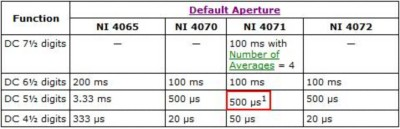Table 1: NI 4071 5½-Digit Resolution Default Aperture Times from DMM Help

Since we are using an NI 4071 and 5½-digit resolution, we can use Table 1 to determine that our aperture time is 500 µs.

1. Next, we need to add in additional noise in error by looking at the graph from the specifications. We can find it on page three of the NI 4071 DMM Specifications page: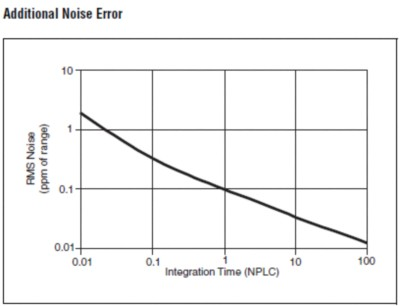Graph 1: Additional Noise Error from 4071 Specifications

Looking at the graph, the RMS Noise is related to the number of power line cycles (NPLC), not seconds. We will convert our aperture time into PLC. In countries that have a mains power frequency of 60 Hz, 1 PLC equals 16.67 ms.  In countries that have a mains power frequency of 50 Hz, 1 PLC equals 20 ms.

We will assume that we're operating in the USA, which uses 60 Hz powerline signals.  From this we can calculate that 500 µs equals 0.03 PLC. (500us / 16.67 ms =  0.03 PLC)

Now, we look at Graph 1 for the RMS Noise that correlates with 0.03 PLC. Please note that both axes are logarithmic. We see that a 0.03 PLC integration time corresponds to just under 1 ppm of RMS noise, but for simplicity's sake in this example we will use 1 ppm of the range.

1. Now we will find the correct multiplier. We will use the table provided on page three of the specifications: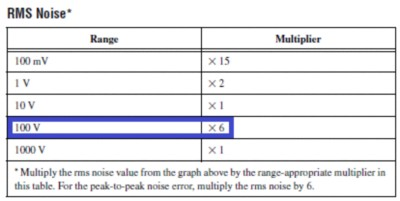Table 2: RMS Noise from 4071 Specifications

As mentioned above, we are interested in the 100 V range so our multiplier is 6. However, we are also interested in the peak-to-peak range, so we need to multiply it by an additional 6. Since accuracy is specified in terms of ±, we actually want half so we will divide by 2. Our multiplier has become 6 x 6 ÷ 2 = 18.

The RMS Noise was 1 ppm of the range. Multiplying this by our multiplier: 1 x 18 = 18 ppm of the range.

1. Lastly, we need to determine our accuracy equation. First, use the accuracy table provided in the specifications in order to get a starting point: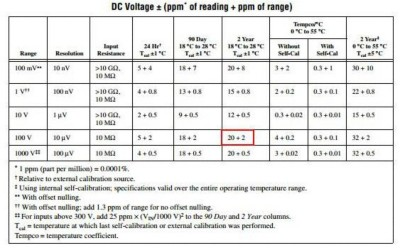Table 3: NI 4071 DC Voltage Accuracy Specifications

We are interested in the 100 V range, our device was last calibrated between 90 days and 2 years ago, and it is within 1°C from the temperature at last calibration. At 7½-digit resolution, our coefficients would be 20 + 2, making our equation be DC Voltage ± (20 ppm of reading + 2 ppm of the range).

We will add the 18 ppm of the range to our range coefficient: 18 ppm + 2 ppm = 20 ppm of the range. Our final equation is DC Voltage ± (20 ppm of reading + 20 ppm of the range).

## NI 4070/4072

The NI 4070 and NI 4072 have identical specifications for most of their measurement functions.  The NI 4072 adds the ability to take Capacitance and Inductance measurements.

For this example, we will assume the following:

• We are using an NI 4070
• Our NI 4070 was externally calibrated between 90 days and 2 years ago
• Our NI 4070 is within 5°C from the temperature at last self-calibration or external calibration
• We are looking for DC voltage accuracy in the 1 V range
• We want to know the accuracy at 5½-digit resolution
• We are using the default aperture time

1. First, you will need to locate the accuracy of the measurement you are taking in the NI 4070/4072 specifications document.  This table shows accuracy levels when programming the device to measure at 6½ digits of resolution.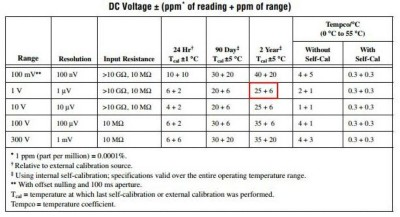Table 4: NI 4070/4072 DC Voltage Accuracy Specifications

Since we are using an NI 4070 in DC Voltage mode in the 1 V range, we can use Table 4 to determine that our starting accuracy value is 25 ppm of reading + 6 ppm of range.

1. Next, we need to add in additional noise in error by looking at the table from the specifications. We can find it on page four of the NI 4070 DMM Specifications and NI 4072 DMM Specifications: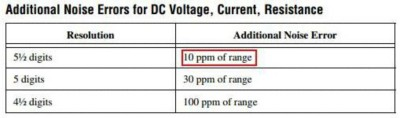Table 5: NI 4070/4072 Additional Noise Errors from the Spec Document

When operating at 5½ digits of resolution, 10 ppm must be added to the offset accuracy spec.

1. We are interested in the 1 V range, our device was last calibrated between 90 days and 2 years ago, and it is within 5°C from the temperature at last calibration. At 6½-digit resolution, our coefficients would be 25 + 6, making our equation be DC Voltage ± (25 ppm of reading + 6 ppm of range).

For 5½-digit resolution, we add the additional 10 ppm of range to our range coefficient: 6 ppm + 10 ppm = 16 ppm of range. Our final accuracy value is DC Voltage ± (25 ppm of reading + 16 ppm of range).

## NI 4065

For this example, we will assume the following:

• Our NI 4065 was externally calibrated between 90 days and 1 year ago
• Our NI 4065 is within 5°C from the temperature at last external calibration
• We are looking for DC voltage accuracy in the 10 V range
• We want to know the accuracy at 5½-digit resolution
• We are using the default aperture time

1. First, you will need to determine the aperture time for your DMM. Default aperture times are documented in NI Digital Multimeters Help » Devices » NI 4065 » DMM Measurements » DMM Measurement Cycle » Aperture Time.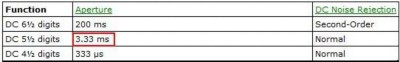Table 6: NI 4065 5½-Digit Resolution Default Aperture Times from DMM Help

Since we are using an NI 4065 and 5½-digit resolution, we can use Table 6 to determine that our aperture time is 3.33 ms.

1. Next, we need to add in additional noise in error by looking at the graph from the specifications. We can find it on page three of the NI 4065 DMM Specifications page: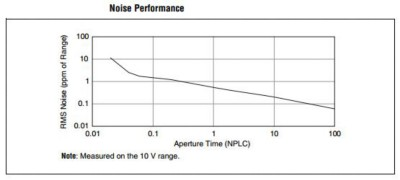Graph 2: Additional Noise Error from 4065 Specifications

Looking at the graph, the RMS Noise is related to the number of power line cycles (NPLC), not seconds. We will convert our aperture time into PLC. In countries that have a mains power frequency of 60 Hz, 1 PLC equals 16.67 ms.  In countries that have a mains power frequency of 50 Hz, 1 PLC equals 20 ms.

We will assume that we're operating in the USA, which uses 60 Hz powerline signals. From this you can calculate that 3.33 ms is equal to 0.2 PLC. (3.33 ms / 16.67 ms = ~0.2 PLC)

Now we look at Graph 2 for the RMS Noise that correlates with 0.2 PLC. Please note that both axes are logarithmic. We see that 0.2 PLC falls right at 1 ppm of RMS noise.

1. Lastly, we need to determine our accuracy equation. First, use the accuracy table provided in the specifications in order to get a starting point: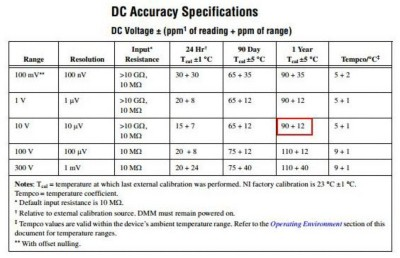Table 7: NI 4065 DC Voltage Accuracy Specifications

Since we are using an NI 4065 in DC Voltage mode in the 10 V range, we can use Table 7 to determine that our starting accuracy value is 90 ppm of reading + 12 ppm of range.

1. We are interested in the 10 V range, our device was last calibrated between 90 days and 1 year ago, and it is within 5°C from the temperature at last external calibration. At 6½-digit resolution, our coefficients would be 90 + 12, making our equation be DC Voltage ± (90 ppm of reading + 12 ppm of the range).

We will add the 1 ppm of the range to our range coefficient: 12 ppm + 1 ppm = 13 ppm of the range. Our final equation is DC Voltage ± (90 ppm of reading + 13 ppm of the range).